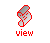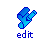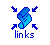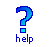## Code Format

While Smalltalk and Java defined virtual machines with dozens of bytecodes, Self traditionally has done its job with only eight (Self 4.1 has added a few more). All of them are designed around the concept of a stack machine, or a zero address machine.

The description of the current code format can be seen in the Oliver page. This is optimized for a hardwired hardware implementation. For example: in software it is cheaper to add than to replace bits but in hardware rearranging bits is just wires and so extremely cheap.

All addressing is done relative to the stack top rather than some frame base, so the memory bandwidth for saving and restoring frame pointers is saved. This requires the compiler to adjust addresses as the stack grows and shrinks and the return instruction must know how much to trim back the stack to eliminate the current frame.

Another optimization that makes the stack simpler in the most common case is the scheme adopted in VisualWorks 5 and Squeak VI4 where blocks don't directly access previous frames. Rather they copy any read-only information they need and store any shared information in special indirection arrays. The example given in the VisualWorks paper is:

inject: thisValue into: binaryBlock
```    |nextValue|
nextValue := thisValue.
self do: [ :each | nextValue := binaryBlock value: nextValue value: each].
^nextValue
```
This has one shared mutable data (nextValue) and one shared read-only data (binaryBlock). By using an indirection array both shared data become read-only:

inject: thisValue int: binaryBlock
```    |indVec|
indVec := Array new: 1 with: thisValue.
self do: [ :each | indVec at: 1 put: (binaryBlock value: (indVec at: 1) value: each)].
^indVec at: 1
```
Using regular array operations this would be a bit slow and long, so a small set of special instructions take care of the indirections. We translate the main method to these bytecodes:

 bytecode description stack stack stack stack stack stack stack . inject:into: thisValue binaryBlock 90 tmp 0 thisValue thisValue binaryBlock 12 const 1 1 thisValue thisValue binaryBlock 3E create array indVec thisValue binaryBlock 92 tmp 1 binaryBlock indVec thisValue binaryBlock 91 tmp 2 indVec binaryBlock indVec thisValue binaryBlock 87 literal valuePC indVec binaryBlock indVec thisValue binaryBlock 14 const 2 2 valuePC indVec binaryBlock indVec thisValue binaryBlock 2F block newBlock indVec thisValue binaryBlock 3C push self self newBlock indVec thisValue binaryBlock 48 send #do: result indVec thisValue binaryBlock D0 tmpPut #0 (pop) indVec thisValue binaryBlock A0 ind 2 nextValue indVec thisValue binaryBlock C3 return #3 nextValue ?? ?? ?? valuePC ?? ?? #do:

The code for the block is translated to:

 bytecode description stack stack stack stack stack stack stack . value: newBlock each A0 ind 0 indVec newBlock each 00 A2 ind 0,1 binaryBlock indVec newBlock each 93 tmp 1 each binaryBlock indVec newBlock each A2 ind 2 nextValue each binaryBlock indVec newBlock each 92 tmp 3 binaryBlock nextValue each binaryBlock indVec newBlock each 45 send #value:value: result binaryBlock indVec newBlock each D2 indPut 2 binaryBlock indVec newBlock each A1 ind 2 result binaryBlock indVec newBlock each C4 return #4 result ?? ?? #value:value:

The numbers in the descriptions (like var 2) refer to what the original Smalltalk bytecodes would use. Not only does the varying stack complicate the mapping to what the actual bytecode uses, but the fact that temporaries grow in the opposite direction that arguments adds to the confusion:

 stack stack stack stack tmp 0 tmp 1 tmp 2 tmp 0 tmp 1 tmp 3 tmp 2 tmp 0 tmp 1

A copy of "self" is present in the return stack at a known depth and a special bytecode can fetch it when needed.

The compiler isn't very smart so the above code has a few redudant instructions, like fetching "binaryBlock" from the block data into a temporary even though that is only used once.

Note that this example is the extreme case and most code wouldn't have all these indirections and copying.

# Smalltalk-80 ("Blue Book")

The original specification for the Smalltalk virtual machine tried to have a very compact encoding with a basic instruction length of one byte. This is described in detail in chapter 28 of the book "Smalltalk-80: The Language And Its Implementation" by Adele Goldberg and David Robson. This was known as the "blue book" and the current version ("purple book") no longer includes the chapters about the implementation, but fortunately these are
avaliable online.

# Previous Design (presented at OOPSLA 2003)

Everything is described in terms of objects and messages. The hardware defines a set of "primitive objects", each of which can understand two distinct messages. The basic bytecode format is

 r r r m o o o o

where "rrr" indicates one of the eight primitive objects as the receiver, "m" is the message that is sent to it and "oooo" is a four bit argument.

Two special registers hold copies of the top elements of the stack: T and S. The stack pointer indicates the memory position corresponding to S.

 receiver message description operation 000 = direct 0 = send: the argument is the message to be sent see the next table 1 = push: the argument is pushed on the stack T:=op , S:=T , r[sp]:=S , sp:=sp+ 001 = indirect 0 = send:to: the argument is an index into the literal vector and indicates the selector of the message to be sent to the object on the top of the stack (TOS) m:=map(T) , p:=vpc/\mask + op<<2 , z:=0while(m!=z) {k:=icache[p] , p:=p+x:=icache[k] , k:=k+}vpc:=k 1 = push: the argument is an index into the literal vector and indicates what should be pushed on the stack T:=icache[vpc/\mask + (op<<2)] , S:=T , r[sp]:=S , sp:=sp+ 010 = state 0 = read:in: the argument selects a field from the object on TOS and its contents replace the TOS T:=mem[T:op] 1 = write:in:with: the argument selects a field in the object on TOS, which is replaced by the elemment next to the top of stack. The two elements are eliminated from the stack mem[T:op]:=S , T:=r[sp-] , sp:=sp-S:=r[sp-] , sp:=sp- 011 = frame 0 = read: the argument selects a field from the (normally) current frame and its contents are pushed on the stack. If the argument is larger than 15 (using the extension instructions below) then the top bits indicate the number of lexical levels to "walk" to get to the actual frame n:=op>>4 , i:=op/\15 , f:=spwhile(n>0) {f:=r[f,3] , n:=n-}T:=r[f:i] , S:=T, r[sp]:=S , sp:=sp+ 1 = write:with: the argument selects a field in the (normally) current frame, which is replaced by the TOS. The TOS is eliminated n:=op>>4 , i:=op/\15 , f:=spwhile(n>0) {f:=r[f,3] , n:=n-}r[f:i]:=T , T:=S , S:=r[sp-] , sp:=sp- 100 = stream 0 = next: the argument selects two fields in the current frame. The first indicates an object and the second a field inside that object which is pushed on the stack. The pair is always aligned so that the first is the even one and if the argument is odd, then the index is incremented. In other words: an argument of 6 selects field 6 as the object pointer and field 7 as the index while an argument of 7 selects the same two "registers" but automatically increments field 7 p:=op/\14T:=mem[r[p]:r[p+1]] , S:=T , r[sp]:=S , sp:=sp+ , if(op/\1){r[p+1]:=r[p+1]+} 1 = next:put: the argument selects two fields in the current frame as above, which indicate a given field in an object. The TOS ir written there and eliminated p:=op/\14mem[r[p]:r[p+1]]:=T , T:=S , S:=r[sp-] , sp:=sp- , if(op/\1){r[p+1]:=r[p+1]+} 101 0 1 110 = jump 0 = to:onZero: the argument replaces the bottom bits of the instruction pointer, but only if the TOS is zero. The TOS is eliminated in any case if (T==0) {vpc:=vpc/\mask + op} , T:=S , S:=r[sp-] , sp:=sp- 1 = always: the argument replaces the bottom bits of the instruction pointer vpc:=vpc/\mask + op 111 = extend 0 = positive: the argument is used to add four more bits to the argument of the next instruction op:=op<<4 + ir/\15 1 = negative: the argument is used to add four more bits to the argument of the next instructions, but the top bits are all set op:=-16 + ir/\15

Operations marked in red might cause a trap to the stack cache overflow/underflow software. Operations in green might cause a second level icache miss, which is handled in software (the first level miss is handled by the hardware). Operations in blue might cause a data cache miss, which is usually handled by hardware but might trap to software when the object is not found (either its segment isn't loaded or it only exists in a compressed state).

In addition to the operations shown above, all instructions except the extend ones start with op:=op<<4+ir/\15 and end with op:=0. All instructions except jump and indirect send end with vpc:=pc+. There is an extra fetch cycle that does ir:=icache[vpc]. The "mask" that appears in some instructions depends on the current size of op either as is or converted from words to bytes, depending on the instruction.

The send: and send:to: instructions are particularly interesting because they add a level of indirection. The latter is the regular high level message send and expresses most of the elements in the original source code. The direct send: is interpreted as also sending a one bit message to a selected primitive object but without the extra argument of the basic bytecodes. And the "address space" of these primitive objects is separate from the one in the basic bytecodes (and isn't limited to three bits, thanks to the extend bytecodes), as shown in the following table:

 receiver message description operation 000 = stack 0 = dup: makes a copy of the TOS S:=T , r[sp]:=S , sp:=sp+ 1 = pop: eliminates the TOS T:=S , S:=r[sp-] , sp:=sp- 001 = end 0 = returnFar: unwinds the stack of frames to the outermost frame in which the current one is lexically embedded and transfers the current TOS there while(r)do{sp:=r}sp:=rS:=r[sp] 1 = returnNear: unwinds the stack of frames one level and transfers the current TOS there sp:=rS:=r[sp] 010 = context 0 = new allocates a new frame in the stack cache and initializes the first few fields r[sp]:=S , r[sp+]:=Tn:=r[0,2]r[n,0]:=n , r[n,1]:=vpc , r[n,2]:=sp , r[n,3]:=0sp:=n-S:=r[sp] , T:=r[sp+] 1 = grabArg the TOS in the previous level is transferred to the current one S:=T , r[sp+]:=T , sp:=sp+n:=rT:=r[n]r[n,0]:=r[n,0]- 011 0 1 100 = rawInteger 0 = add:with: replaces the top two elements on the stack with TOS+NOS T:=T+S , S:=r[sp-] , sp:=sp- 1 = subtract:from: replaces the top two elements on the stack with TOS-NOS T:=T-S , S:=r[sp-] , sp:=sp- 101 = shifter 0 = left: multiplies TOS by two T:=T<<1 1 = right: divides TOS by two T:=T>>1 110 = bits 0 = and:with: replaces the top two elements on the stack with TOS/\NOS T:=T/\S , S:=r[sp-] , sp:=sp- 1 = or:with: replaces the top two elements on the stack with TOS\/NOS T:=T\/S , S:=r[sp-] , sp:=sp- 111 = compare 0 = signed:with: replaces the top two elements on the stack an indication of the relative magnitudes of TOS and NOS T:=TS?1:0) , S:=r[sp-] , sp:=sp- 1 = xor:with: replaces the top two elements on the stack with TOS^NOS T:=T^S , S:=r[sp-] , sp:=sp-

Only 28 of the possible 31 "short instructions" have been defined so far. All 256 instructions are shown in this table (the most significant bits are in the lines):

It should be obvious that several of these instructions are a really bad idea or don't make sense, and so should never be executed. They are marked in red in the above table. The ones marked in blue would be ok except that word 0 in each eight word block in the instruction cache is reserved for the vpc value for that block. Both kinds of instructions are there to make the project more uniform and simpler.

It would seem that an instruction such as "ext 0" would have no effect, but it actually changes the mask value for the following instruction and that could change its meaning. Any instruction not defined above is considered a nop (no operation).

# Self

Self 4.1.2 uses four bits for the op code field and four bits for the index field:

 opcode name description 0 index extend the index field of the next bytecode 1 literal push the literal onto the top of stack (tos) 2 send send the message with the literal as selector and tos as receiver 3 implicitSelfSend send the message to self with the literal as selector 4 extended see below 5 readLocal access local slot 6 writeLocal change value of local slot 7 lexicalLevel change what "local" means for previous instructions 8 branchAlways jump to indicated bytecode (literal must be smallInt) 9 branchIfTrue only jump if tos == true 10 branchIfFalse only jump if tos == false 11 branchIndexed tos is an index into a "branch vector" 12 delegatee changes the next "send" into a directed resend 13 undefined 14 undefined 15 undefined

For the extended instructions (opcode 4) the index field has the actual opcode:

 index name description 0 pushSelf puts the current receiver on the tos 1 pop eliminates the tos 2 nonLocalReturn returns from this block's "home context" 3 undirectedResend like "super" in Smalltalk

In Self 4.1 and older, there were only 8 bytecodes. The bottom five bits were the operand and the top three bits were the opcodes:

 index name description 0 extend extends the operand of the next instruction with its own operand 1 self pushes the receiver on the stack (ignores operand) 2 literal pushes the value in the literal vector indexed by its operand on the stack 3 non local return returns from the most external lexical context with the value on the top of the stack (ignores operand) 4 directee selects a specific parent for the following instruction, which must be a resend 5 send sends a message to the object on the top of the stack with the literal indexed by the operand as the selector 6 implicit self send sends a message to the current receiver (lookup actually starts in the local context) with the literal indexed by the operand as the selector 7 resend like "super" in Smalltalk

# Older Alternatives

Is it possible to have even fewer than 8 instructions? Well, two of the Self bytecodes implement regular and directed resends (messages to super, in Smalltalk terms) but they are so rarely used that they could be handled as primitives (which use the regular send bytecodes in Self) without much overhead. And the "push self" bytecode is redundant since each method context has a slot named "self" and so sending a message with this selector to it would yield the exact same result.

The "extend index" bytecode is only needed because of the small size of the literal index field in the bytecode (5 bits allows at most 32 literals per methods). If we were to match each instruction directly with its literal operand we could drop this bytecode and would need only 2 bits to encode the remaining four instructions. The problem is that now literals which appear repeatedly in the source will no longer use up a single literal vector entry. Surprisingly, there is a net saving of space with this approach. See:

http://groups.yahoo.com/group/self-interest/message/299

Can we continue this trimming even further? Sure, if we don't mind some more radical changes in the virtual machine. First we allow slots to contain not only regular objects and methods but "continuations" as well. For more about continuations, see the Lisp dialect Scheme. Now when we create a new context object, not only do we include a "self" slot filled with the receiver but also a "^" slot pointing to the sending context (directly or suitably wrapped if we need to distinguish contexts from continuations). Sending the up arrow message ("^" is the poor ASCII approximation), with some expression as an argument, to the current context (an implicit self send) will give us the exact effect of a non local return in Self or Smalltalk. Simply not having these slots for block contexts (they would inherit them from their outer context) does the right thing automatically.

Now all we have left is message sends and "push literal". Most objects can't be used as message selectors and we could modify that to "no objects can be used" by creating a special selector type instead of using regular immutable strings. Such a change could be a good idea in any case for the persistent object system. I'll take an example from the Smalltalk "blue book":

```merge: aRectangle
| minPoint maxPoint |
minPoint <- origin min: aRectangle origin.
maxPoint <- corner max: aRectangle corner.
^ Rectangle origin: minPoint
corner: maxPoint```

and rewrite it as Self:

```merge: aRectangle = ( | minPoint. maxPoint |
minPoint: origin min: aRectangle origin.
maxPoint: corner max: aRectangle corner.
Rectangle origin: minPoint Corner: maxPoint
)```

Note that there are no literals here (see the part about syntax for more on that) and there is no need to have an up arrow before the last expression (though it would still work if there was one). We still need one bit to distinguish between regular message sends and implicit receiver message sends, so I will use color for that. Green selectors will be sent to the top of the stack while red selectors will be sent to the current context. Vectors will be shown as space separated elements between parenthesis, and code will just be a vector of selectors:

```  ( aRectangle origin origin min: minPoint:
aRectangle corner corner max: maxPoint:
maxPoint minPoint Rectangle origin:Corner: )```

Due to a lack of "pop" or "drop" instructions, this method will accumulate useless stuff on the stack as it executes but that is not really a problem. This looks a lot like Forth:

`     arguments receiver selector`

We might make it more Lisp-like instead by reversing that order. An interesting alternative would be an infix notation similar to that used in the source:

`     receiver selector arguments`

So our vm level code for the example method would look like:

```( ( minPoint: ( ( origin ) min: ( aRectangle origin ) ) )
( maxPoint: ( ( corner ) max: ( aRectangle corner ) ) )
( ( Rectangle ) origin:Corner: ( minPoint ) ( maxPoint ) ) )```

We don't need colors to separate the regular and implicit receiver messages since the latter are always the first selector in each subvector while the former are always the second element.

This is close enough to an Abstract Syntax Tree (AST) representation of the code that it is easy to do all kinds of code manipulation. It isn't too easy to read, however, and the large number of nested vector implies a lot of overhead. One simplification is possible since the first selector in an argument expression can be always considered an implied receiver send even if it isn't in a nested vector:

```( ( minPoint: origin min: aRectangle origin )
( maxPoint: corner max: aRectangle corner )
( Rectangle origin:Corner: minPoint ( maxPoint ) ) )```

This is harder to manipulate since it is closer to the unparsed source, but it is actually just as easy to interpret. One additional step in this direction would be to include a special "." token to separate top level expressions and arguments instead of using nested vectors:

```( minPoint: origin min: aRectangle origin .
maxPoint: corner max: aRectangle corner .
Rectangle origin:Corner: minPoint . maxPoint )```

And so we are back to a token stream format for virtual machine code, just like what the very first Smalltalk implementations used. Though this last format is the most compact (after the Forth-like one), the more structured one above it might be the best choice.